Calculate importance scores for planning units selected in a solution based on the replacement cost method (Cabeza and Moilanen 2006).

eval_replacement_importance(x, solution, ...)

# S4 method for ConservationProblem,numeric
eval_replacement_importance(x, solution, rescale, run_checks, force, threads, ...)

# S4 method for ConservationProblem,matrix
eval_replacement_importance(x, solution, rescale, run_checks, force, threads, ...)

# S4 method for ConservationProblem,data.frame
eval_replacement_importance(x, solution, rescale, run_checks, force, threads, ...)

# S4 method for ConservationProblem,Spatial
eval_replacement_importance(x, solution, rescale, run_checks, force, threads, ...)

# S4 method for ConservationProblem,sf
eval_replacement_importance(x, solution, rescale, run_checks, force, threads, ...)

# S4 method for ConservationProblem,Raster
eval_replacement_importance(x, solution, rescale, run_checks, force, threads, ...)

## Arguments

x

problem() (i.e., ConservationProblem) object.

solution

numeric, matrix, data.frame, Raster, Spatial, or sf::sf() object. The argument should be in the same format as the planning unit cost data in the argument to x. See the Solution format section for more information.

...

not used.

rescale

logical flag indicating if replacement cost values---excepting infinite (Inf) and zero values---should be rescaled to range between 0.01 and 1. Defaults to TRUE.

run_checks

logical flag indicating whether presolve checks should be run prior solving the problem. These checks are performed using the presolve_check() function. Defaults to TRUE. Skipping these checks may reduce run time for large problems.

force

logical flag indicating if an attempt to should be made to solve the problem even if potential issues were detected during the presolve checks. Defaults to FALSE.

integer number of threads to use for the optimization algorithm. Defaults to 1 such that only a single thread is used.

## Value

A numeric, matrix, data.frame

RasterLayer, Spatial, or sf::sf() object containing the importance scores for each planning unit in the solution. Specifically, the returned object is in the same format as the planning unit data in the argument to x.

## Details

This function implements a modified version of the replacement cost method (Cabeza and Moilanen 2006). Specifically, the score for each planning unit is calculated as the difference in the objective value of a solution when each planning unit is locked out and the optimization processes rerun with all other selected planning units locked in. In other words, the replacement cost metric corresponds to change in solution quality incurred if a given planning unit cannot be acquired when implementing the solution and the next best planning unit (or set of planning units) will need to be considered instead. Thus planning units with a higher score are more important (and irreplaceable). For example, when using the minimum set objective function (add_min_set_objective()), the replacement cost scores correspond to the additional costs needed to meet targets when each planning unit is locked out. When using the maximum utility objective function (add_max_utility_objective(), the replacement cost scores correspond to the reduction in the utility when each planning unit is locked out. Infinite values mean that no feasible solution exists when planning units are locked out---they are absolutely essential for obtaining a solution (e.g., they contain rare species that are not found in any other planning units or were locked in). Zeros values mean that planning units can swapped with other planning units and this will have no effect on the performance of the solution at all (e.g., because they were only selected due to spatial fragmentation penalties).

These calculations can take a long time to complete for large or complex conservation planning problems. As such, we using this method for small or moderate-sized conservation planning problems (e.g., < 30,000 planning units). To reduce run time, we recommend calculating these scores without additional penalties (e.g., add_boundary_penalties()) or spatial constraints (e.g., add_contiguity_constraints()). To further reduce run time, we recommend using proportion-type decisions when calculating the scores (see below for an example).

## Solution format

Broadly speaking, the argument to solution must be in the same format as the planning unit data in the argument to x. Further details on the correct format are listed separately for each of the different planning unit data formats:

x has numeric planning units

The argument to solution must be a numeric vector with each element corresponding to a different planning unit. It should have the same number of planning units as those in the argument to x. Additionally, any planning units missing cost (NA) values should also have missing (NA) values in the argument to solution.

x has matrix planning units

The argument to solution must be a matrix vector with each row corresponding to a different planning unit, and each column correspond to a different management zone. It should have the same number of planning units and zones as those in the argument to x. Additionally, any planning units missing cost (NA) values for a particular zone should also have a missing (NA) values in the argument to solution.

x has Raster planning units

The argument to solution be a Raster object where different grid cells (pixels) correspond to different planning units and layers correspond to a different management zones. It should have the same dimensionality (rows, columns, layers), resolution, extent, and coordinate reference system as the planning units in the argument to x. Additionally, any planning units missing cost (NA) values for a particular zone should also have missing (NA) values in the argument to solution.

x has data.frame planning units

The argument to solution must be a data.frame with each column corresponding to a different zone, each row corresponding to a different planning unit, and cell values corresponding to the solution value. This means that if a data.frame object containing the solution also contains additional columns, then these columns will need to be subsetted prior to using this function (see below for example with sf::sf() data). Additionally, any planning units missing cost (NA) values for a particular zone should also have missing (NA) values in the argument to solution.

x has Spatial planning units

The argument to solution must be a Spatial object with each column corresponding to a different zone, each row corresponding to a different planning unit, and cell values corresponding to the solution value. This means that if the Spatial object containing the solution also contains additional columns, then these columns will need to be subsetted prior to using this function (see below for example with sf::sf() data). Additionally, the argument to solution must also have the same coordinate reference system as the planning unit data. Furthermore, any planning units missing cost (NA) values for a particular zone should also have missing (NA) values in the argument to solution.

x has sf::sf() planning units

The argument to solution must be a sf::sf() object with each column corresponding to a different zone, each row corresponding to a different planning unit, and cell values corresponding to the solution value. This means that if the sf::sf() object containing the solution also contains additional columns, then these columns will need to be subsetted prior to using this function (see below for example). Additionally, the argument to solution must also have the same coordinate reference system as the planning unit data. Furthermore, any planning units missing cost (NA) values for a particular zone should also have missing (NA) values in the argument to solution.

See importance for an overview of all functions for evaluating the importance of planning units selected in a solution.

Other importances: eval_ferrier_importance(), eval_rare_richness_importance()

## Examples

# \dontrun{
# seed seed for reproducibility
set.seed(600)

data(sim_pu_raster, sim_features, sim_pu_zones_stack, sim_features_zones)

# create minimal problem with binary decisions
p1 <- problem(sim_pu_raster, sim_features) %>%
add_default_solver(gap = 0, verbose = FALSE)
# solve problem
s1 <- solve(p1)

# print solution
print(s1)
#> class      : RasterLayer
#> dimensions : 10, 10, 100  (nrow, ncol, ncell)
#> resolution : 0.1, 0.1  (x, y)
#> extent     : 0, 1, 0, 1  (xmin, xmax, ymin, ymax)
#> crs        : NA
#> source     : memory
#> names      : layer
#> values     : 0, 1  (min, max)
#>

# plot solution
plot(s1, main = "solution", axes = FALSE, box = FALSE)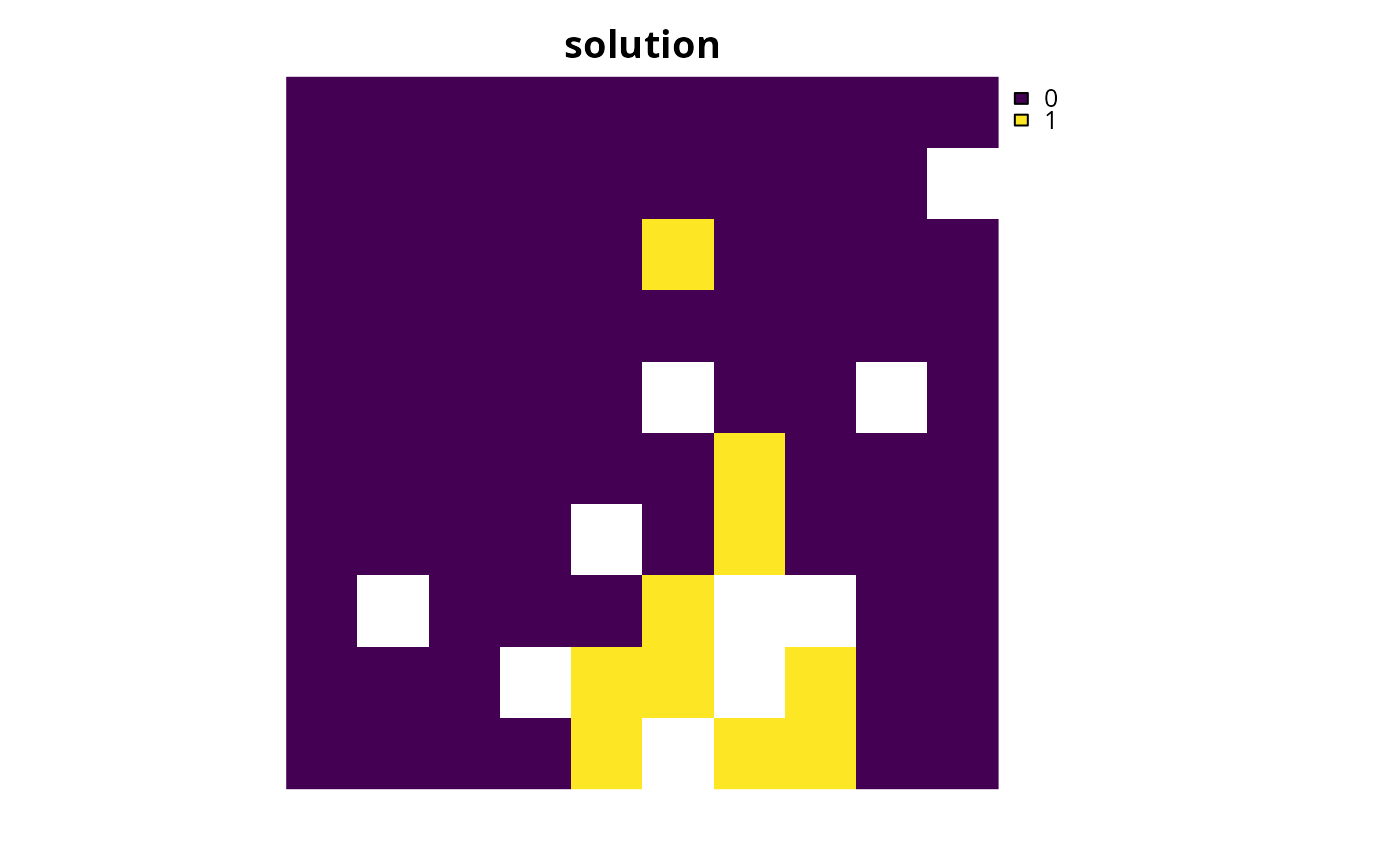# calculate importance scores
rc1 <- eval_replacement_importance(p1, s1)

# print importance scores
print(rc1)
#> class      : RasterLayer
#> dimensions : 10, 10, 100  (nrow, ncol, ncell)
#> resolution : 0.1, 0.1  (x, y)
#> extent     : 0, 1, 0, 1  (xmin, xmax, ymin, ymax)
#> crs        : NA
#> source     : memory
#> names      : rc
#> values     : 0, 1  (min, max)
#>

# plot importance scores
plot(rc1, main = "replacement cost", axes = FALSE, box = FALSE)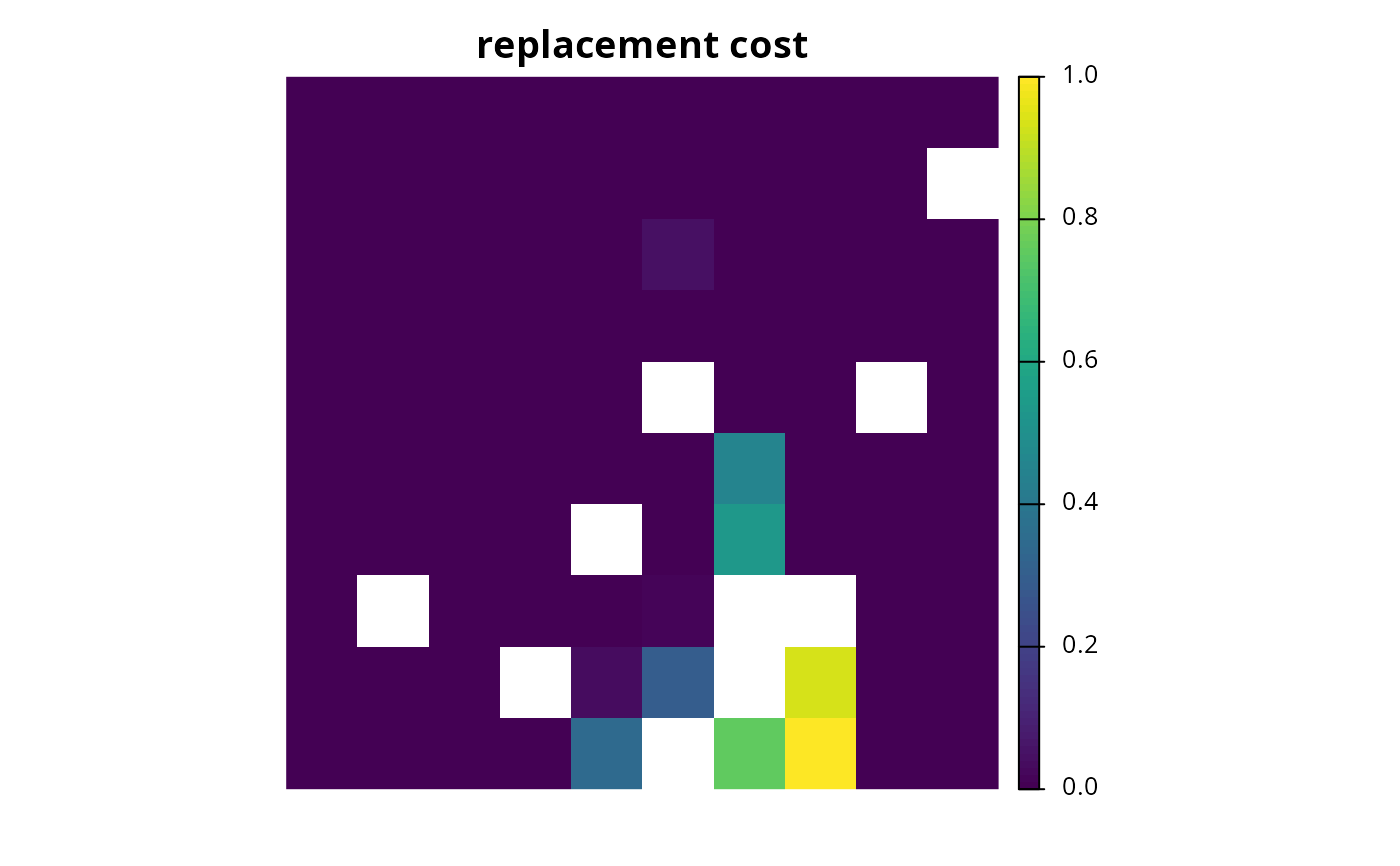# since replacement cost scores can take a long time to calculate with
# binary decisions, we can calculate them using proportion-type
# decision variables. Note we are still calculating the scores for our
# previous solution (s1), we are just using a different optimization
# problem when calculating the scores.
p2 <- problem(sim_pu_raster, sim_features) %>%
add_default_solver(gap = 0, verbose = FALSE)

# calculate importance scores using proportion type decisions
rc2 <- eval_replacement_importance(p2, s1)

# print importance scores based on proportion type decisions
print(rc2)
#> class      : RasterLayer
#> dimensions : 10, 10, 100  (nrow, ncol, ncell)
#> resolution : 0.1, 0.1  (x, y)
#> extent     : 0, 1, 0, 1  (xmin, xmax, ymin, ymax)
#> crs        : NA
#> source     : memory
#> names      : rc
#> values     : 0, 1  (min, max)
#>

# plot importance scores based on proportion type decisions
# we can see that the importance values in rc1 and rc2 are similar,
# and this confirms that the proportion type decisions are a good
# approximation
plot(rc2, main = "replacement cost", axes = FALSE, box = FALSE)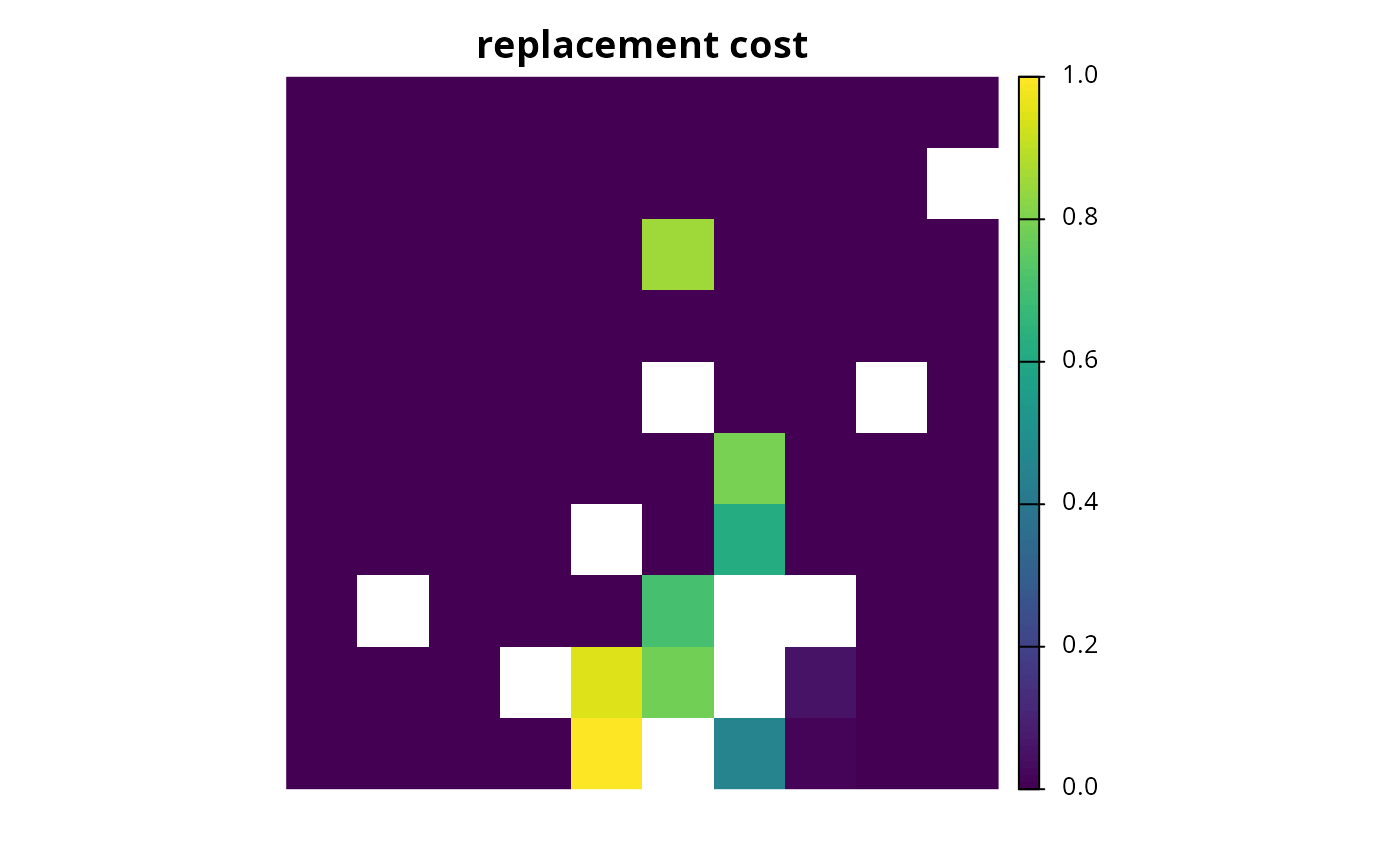# create minimal problem with polygon (sf) planning units
p3 <- problem(sim_pu_sf, sim_features, cost_column = "cost") %>%
add_default_solver(gap = 0, verbose = FALSE)

# solve problem
s3 <- solve(p3)

# print solution
print(s3)
#> Simple feature collection with 90 features and 4 fields
#> Geometry type: POLYGON
#> Dimension:     XY
#> Bounding box:  xmin: 0 ymin: 0 xmax: 1 ymax: 1
#> CRS:           NA
#> First 10 features:
#>        cost locked_in locked_out solution_1                       geometry
#> 1  215.8638     FALSE      FALSE          0 POLYGON ((0 1, 0.1 1, 0.1 0...
#> 2  212.7823     FALSE      FALSE          0 POLYGON ((0.1 1, 0.2 1, 0.2...
#> 3  207.4962     FALSE      FALSE          0 POLYGON ((0.2 1, 0.3 1, 0.3...
#> 4  208.9322     FALSE       TRUE          0 POLYGON ((0.3 1, 0.4 1, 0.4...
#> 5  214.0419     FALSE      FALSE          0 POLYGON ((0.4 1, 0.5 1, 0.5...
#> 6  213.7636     FALSE      FALSE          0 POLYGON ((0.5 1, 0.6 1, 0.6...
#> 7  210.4612     FALSE      FALSE          0 POLYGON ((0.6 1, 0.7 1, 0.7...
#> 8  211.0424     FALSE       TRUE          0 POLYGON ((0.7 1, 0.8 1, 0.8...
#> 9  210.3878     FALSE      FALSE          0 POLYGON ((0.8 1, 0.9 1, 0.9...
#> 10 204.3971     FALSE      FALSE          0 POLYGON ((0.9 1, 1 1, 1 0.9...

# plot solution
plot(s3[, "solution_1"], main = "solution")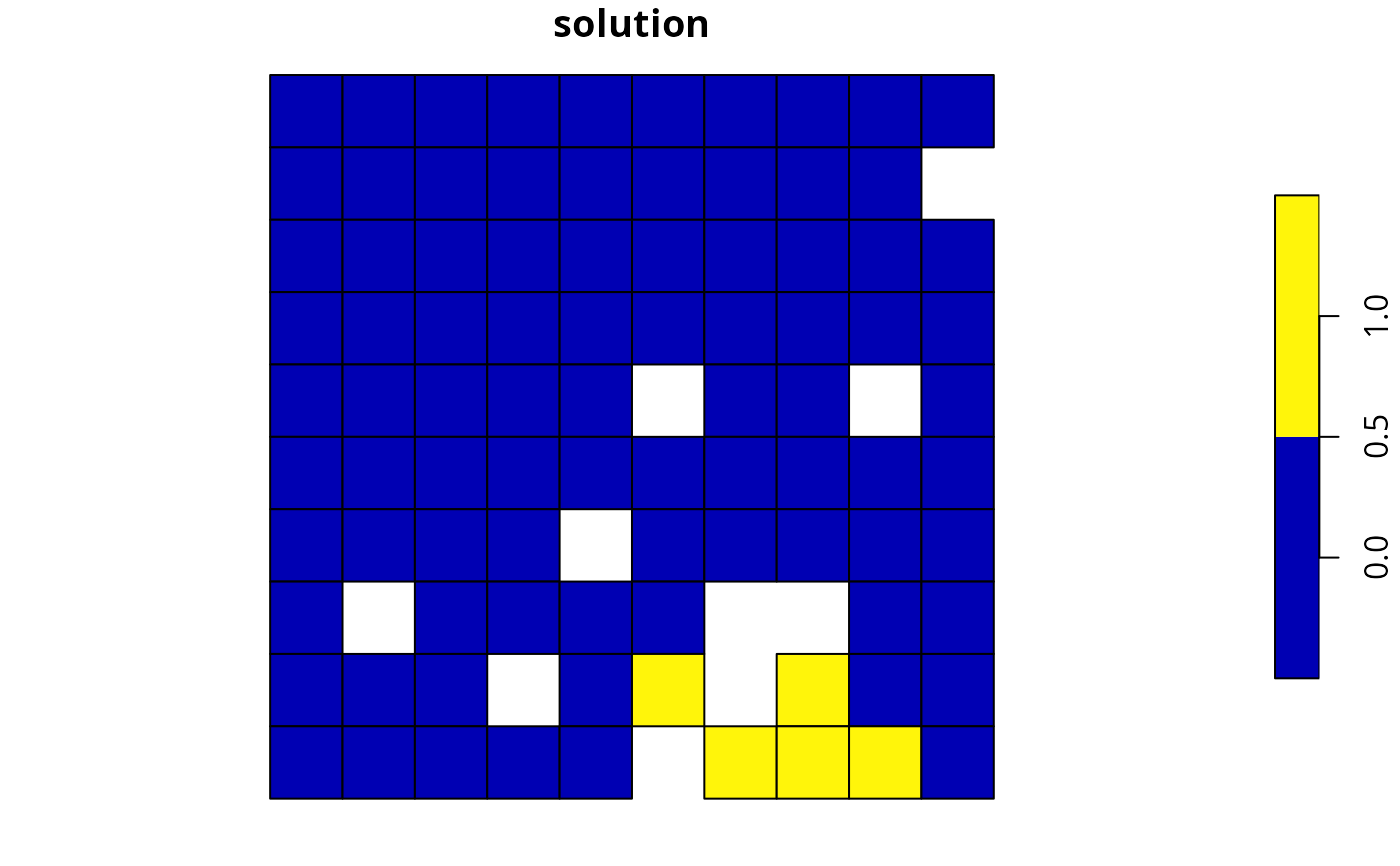# calculate importance scores
rc3 <- eval_rare_richness_importance(p3, s3[, "solution_1"])

# plot importance scores
plot(rc3, main = "replacement cost")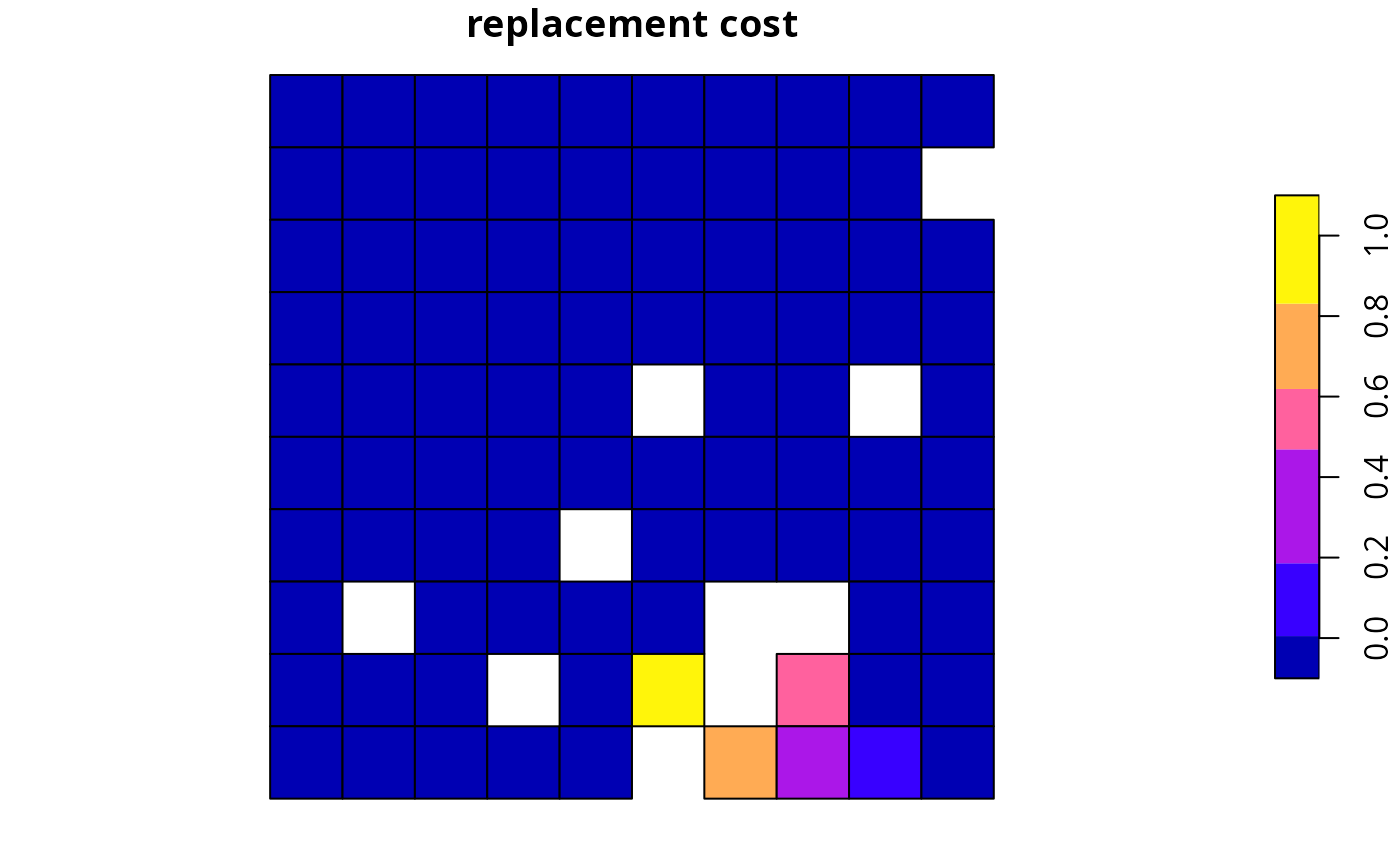# build multi-zone conservation problem with raster data
p4 <- problem(sim_pu_zones_stack, sim_features_zones) %>%
add_relative_targets(matrix(runif(15, 0.1, 0.2), nrow = 5,
ncol = 3)) %>%
add_default_solver(gap = 0, verbose = FALSE)

# solve the problem
s4 <- solve(p4)

# print solution
print(s4)
#> class      : RasterStack
#> dimensions : 10, 10, 100, 3  (nrow, ncol, ncell, nlayers)
#> resolution : 0.1, 0.1  (x, y)
#> extent     : 0, 1, 0, 1  (xmin, xmax, ymin, ymax)
#> crs        : NA
#> names      : zone_1, zone_2, zone_3
#> min values :      0,      0,      0
#> max values :      1,      1,      1
#>

# plot solution
# each panel corresponds to a different zone, and data show the
# status of each planning unit in a given zone
plot(s4, main = paste0("zone ", seq_len(nlayers(s4))), axes = FALSE,
box = FALSE)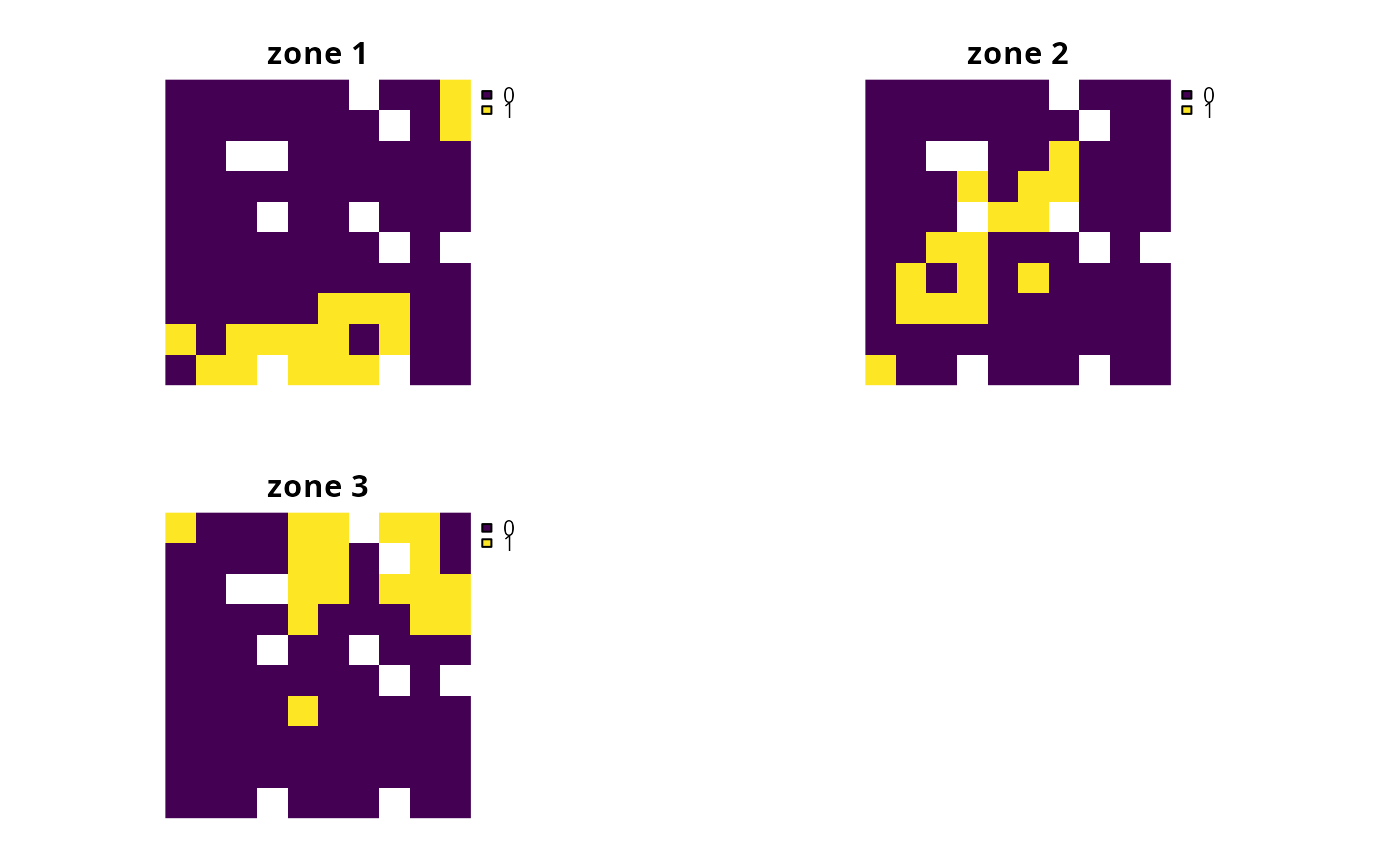# calculate importance scores
rc4 <- eval_replacement_importance(p4, s4)

# plot importance
# each panel corresponds to a different zone, and data show the
# importance of each planning unit in a given zone
plot(rc4, main = paste0("zone ", seq_len(nlayers(s4))), axes = FALSE,
box = FALSE)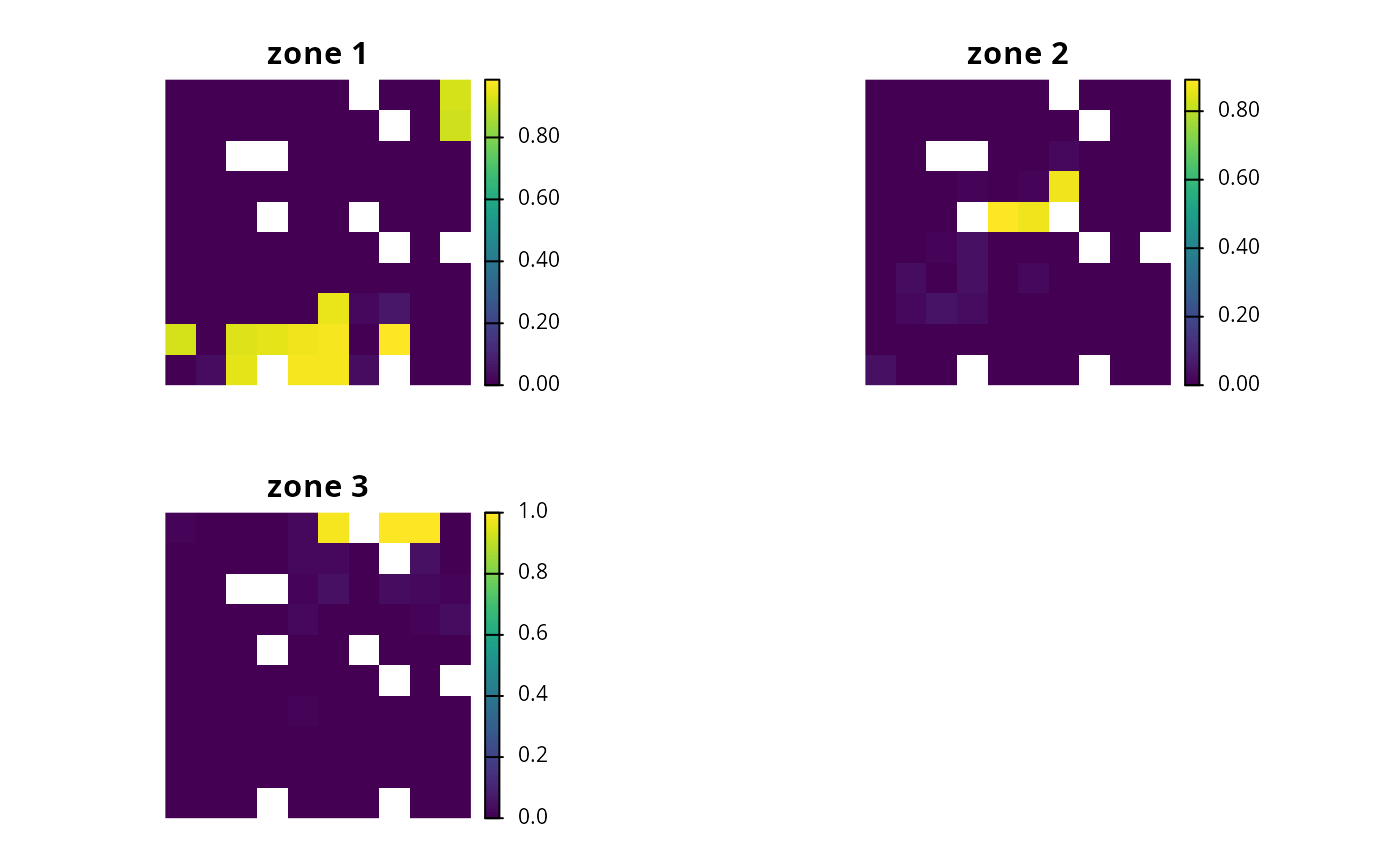# }